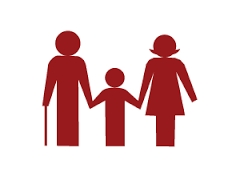# MO Z8-I-1 2018

Fero and David meet daily in the elevator. One morning they found that if they multiply their current age, they get 238. If they did the same after four years, this product would be 378. Determine the sum of the current ages of Fero and David.

Correct result:

s =  31

#### Solution:

$a \cdot \ (s-a)=238 \ \\ (a+4) \cdot \ (s-a+4)=378 \ \\ \ \\ as-a^2=238 \ \\ as-a^2+4a +4s-4a+16=378 \ \\ \ \\ as-a^2=238 \ \\ as-a^2+4s+16=378 \ \\ \ \\ \ \\ 238 + 4s + 16=378 \ \\ \ \\ 238 + 4 \cdot \ s + 16=378 \ \\ \ \\ 4s=124 \ \\ \ \\ s=31$

The equations have the following integer solutions:
a*(s-a)=238
(a+4)*(s-a+4) = 378

Number of solutions found: 2
##### a1=14, s1=31a2=17, s2=31

Calculated by our Diofant problems and integer equations.We would be pleased if you find an error in the word problem, spelling mistakes, or inaccuracies and send it to us. Thank you!Tips to related online calculators
Looking for help with calculating roots of a quadratic equation?
Do you have a linear equation or system of equations and looking for its solution? Or do you have quadratic equation?
Do you solve Diofant problems and looking for a calculator of Diofant integer equations?
Do you want to convert time units like minutes to seconds?

## Next similar math problems:

• The HotelThe Holiday Hotel has the same number of rooms on each floor. Rooms are numbered with natural numbers sequentially from the first floor, no number is omitted, and each room has a different number. Three tourists arrived at the hotel. The first one was in
• Faces diagonalsIf the diagonals of a cuboid are x, y, and z (wall diagonals or three faces) respectively than find the volume of a cuboid. Solve for x=1.3, y=1, z=1.2
• Two chordsCalculate the length of chord AB and perpendicular chord BC to circle if AB is 4 cm from the center of the circle and BC 8 cm from the center of the circle.
• Geometric progressiobIf the sum of four consective terms of geometric progression is 80 and arithmetic mean of second and fourth term is 30 then find terms?
• GP - three membersThe second and third of a geometric progression are 24 and 12(c+1) respectively, given that the sum of the first three terms of progression is 76 determine value of c
• Rectangular triangleThe lengths of the rectangular triangle sides with a longer leg 12 cm form an arithmetic sequence. What is the area of the triangle?
• Right triangle eq2Find the lengths of the sides and the angles in the right triangle. Given area S = 210 and perimeter o = 70.
• Digit sumThe digit sum of the two-digit number is nine. When we turn figures and multiply by the original two-digit number, we get the number 2430. What is the original two-digit number?
• Cuboid wallsCalculate the volume of the cuboid if its different walls have area of 195cm², 135cm² and 117cm².
• Sales of productsFor 80 pieces of two quality products a total sales is 175 Eur. If the first quality product was sold for n EUR per piece (n natural number) and the second quality product after 2 EUR per piece. How many pieces of the first quality were sold?
• The gardenerThe gardener bought trees for 960 CZK. If every tree were cheaper by 12 CZK, he would have gotten four more trees for the same money. How many trees did he buy?
• SegmentsLine segments 62 cm and 2.2 dm long we divide into equal parts which lengths in centimeters is expressed integer. How many ways can we divide?
• Hyperbola equationFind the hyperbola equation with the center of S [0; 0], passing through the points: A [5; 3] B [8; -10]
• Area and perimeter of rectangleThe content area of the rectangle is 3000 cm2, one dimension is 10 cm larger than the other. Determine the perimeter of the rectangle.
• Isosceles triangleIn an isosceles triangle ABC with base AB; A [3,4]; B [1,6] and the vertex C lies on the line 5x - 6y - 16 = 0. Calculate the coordinates of vertex C.
• Lookout towerHow high is the lookout tower? If each step was 3 cm lower, there would be 60 more of them on the lookout tower. If it was 3 cm higher again, it would be 40 less than it is now.
• An equilateralAn equilateral triangle is inscribed in a square of side 1 unit long so that it has one common vertex with the square. What is the area of the inscribed triangle?